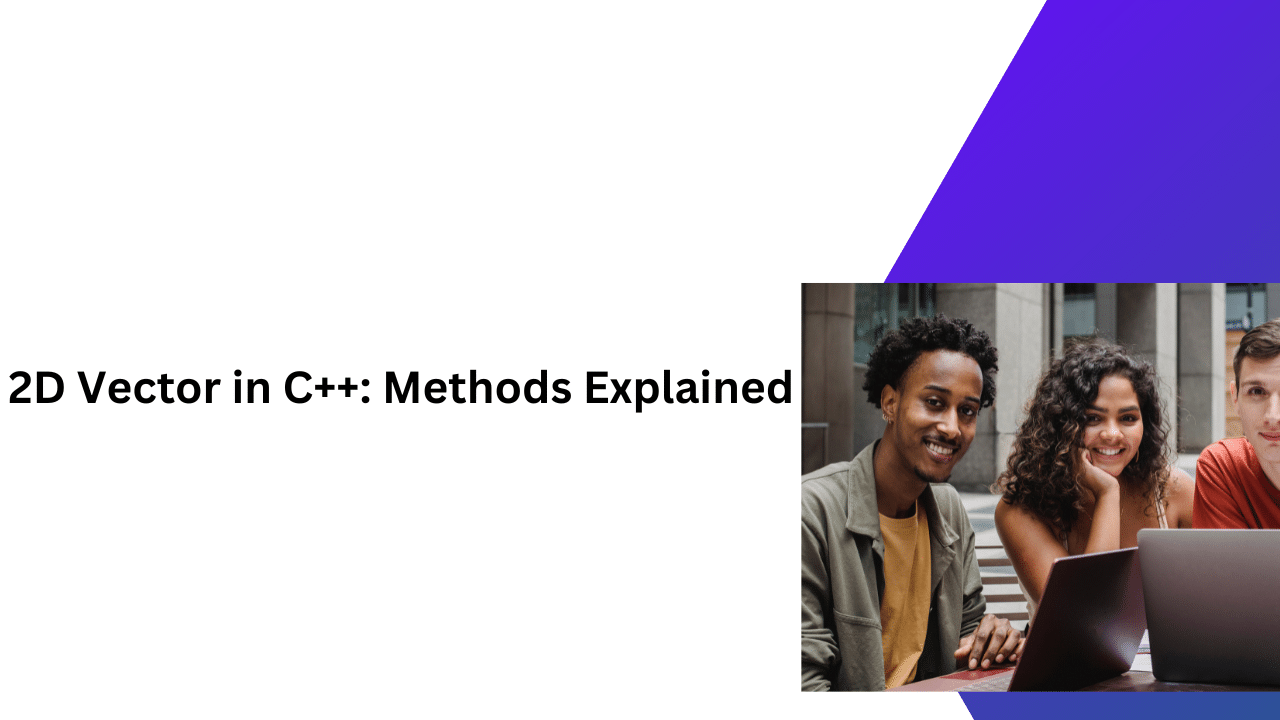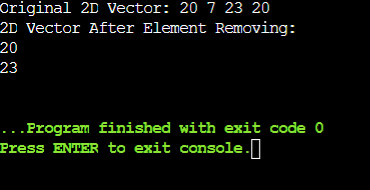# 2D Vector in C++: Methods Explained

You should be aware of the dynamic arrays in different programming languages. But, have you ever thought about the concept of two-dimensional vectors or 2D vectors in C++ programming language?

In this article, all the details of the two-dimensional vector will be discussed along with some very important sample examples. But before we start implementing the sample examples related to two-dimensional vectors, we should know its basic.

So, let us start our discussion.

### What Is A Vector Array In C++ Programming Langauge?

Vector is a special concept in the C++ programming language. It is moreover similar to the array Data Structures concept in different programming languages. But there is an extra feature present. The vector in C++ represents the dynamic properties of elements inside.

``````// Example Of Single Vector Declaration
vector<int> v1 = {1, 2, 3, 4}; // Initializer List Method
vector<int> v2{1, 2, 3, 4}; // Uniform Initialization Method
vector<int> v3(10, 5); // Ten Times 5 Will Add
return 0;``````

That means the vector size can be increased dynamically with the inputs from the user. It is moreover like the linked list where the number of elements can be stretched without losing memory. We should include the vector header file to use the user-defined size.

Did you face any difficulty understanding the code? Don’t worry, you can take help from reliable C++ programming homework experts and clear all your doubts.

### What Is Two Dimensional Vector Or 2D Vector In C++?

A two-dimensional vector is the extended version of a single-dimensional vector. In the two-dimensional vector, two vector concept is used. One is used for making the row & another is used for making the column. It is the same concept just like the 2D array Data Structures in other programming languages.

``````// Example Of Normal 2D Vector Declaration Process
vector<vector<int>> v; // 2D Vector Initialization``````

Just, the difference is that the two-dimensional vector is dynamic. That is the reason, it is more convenient to use. Whenever any elements are deleted or inserted into the 2D vector, it will automatically resize itself.

### What Are The Methods To Declare The 2D Vector In C++?

There are mainly three methods present to declare the 2D vector in C++. One of them has already been demonstrated in the above code snippet. Also, there are two more methods present by which we can declare the 2D vector. Also,  If you’re studying C++, then you must learn about how string declaration is done in C++ to understand the concept of declaration more easily

#### 2D Vector Initialization With Value:

In this case, the 2D vector initialization is done normally, but the values we already provide there. Before moving to the initialization process, the standard template libraries should be included in the program along with the vector header file.

``````// Example Of 2D Vector Declaration Along With Data
#include<vector>
#include<iostream>
using namespace std;

int main()
{
vector<vector<int>> v{{1, 2, 3},{4, 5, 6}};
return 0;
}``````

Here, using the above syntax we will declare a 2D vector int which will collect some values. We will use the braces to indicate the row & column elements inside of the 2D vector.

#### 2D Vector Initialization With Row & Column Values:

In this case, the 2d vector declaration will not be done simply. Here, we don’t need to show the size of the vector using the vector elements. Rather, we will use the row & column numbers to mark the size. Also, the initialization of vectors in C++ can be a little tricky. You can know about the methods used to initialize a vector, there will make your understanding of vectors more easy.

``````// Example Of 2D Vector Declaration Along With Row & Column Data
#include<vector>
#include<iostream>
using namespace std;
int main()
{
int i = 5;
int m = 3;
vector<vector<int>> vec(i, vector<int>(m, 1));
}``````

In the above case, the row & column data are declared in a separate variable. Now, we will use two different vector int concepts to declare the overall 2D vectors in C++ programming language. But it is quite difficult to declare.

### What Is The Process To Traverse Through 2D Vector In C++?

Traversing through the two-dimensional vector is a very simple process to execute. It is like going through the 2D array. Like the 2D array, we should declare the nested for loop to traverse in the 2D vector. But we should declare one first.

``````#include<vector>
#include<iostream>
using namespace std;

int main()
{
vector<vector<int>> zap{{20, 07},{23, 20}}; // Initialization Of Vector Inside Vector
cout << "Traversal Of 2D Vector: " << endl;
for (int i = 0; i < zap.size(); i++) // Outer For Loop For Displaying Vector
{
for (int j = 0; j < zap[i].size(); j++) // Inner Loop For Displaying Vector
cout << zap[i][j] << " ";
cout << endl;
}

return 0;
}``````

In the above code, the inner & outer loops are traversing through the vector & printing out all the data. The same process we will execute for all the upcoming sample examples. The output will show the same thing that we discussed.

### What Are The Major Operations Can Be Done On 2D Vectors In C++?

The above discussion will be enough to clear your basic doubts related to the 2D vectors in C++ programming language. Now, we should understand some of the major operations that can be done on the 2D vectors. They are the following:

1. Insert Vector’s Elements Using push_back() Method
2. Remove Elements Using pop_back() Method

Let us know all of them one by one briefly.

### How To Insert Vector’s Elements Using push_back() Method?

The push_back() is the built-in function present with the standard template library for doing operations on the 2D vector. It is an operation that can be done with the help of another vector. One vector data will be inserted inside of another vector.

The same operation can be done with the default value also. It is like the append operation that adds some data into the empty container one by one. Using the method, in one empty vector, we will insert data from another vector.

General Syntax: new-vector-name.push_back(old-vector-name);

##### Code To Implement Use Of The push_back() Method:
``````#include<vector>
#include<iostream>
using namespace std;

int main()
{
vector<vector<int>> zap; // 2D Vector Initialization
vector<int> one = {20, 07, 20, 23}; // 1D Vector Initialization With Values
zap.push_back(one); // Pushing The Data

cout << "The 2D Vector Data Is: ";
for (int i = 0; i < zap.size(); i++) // Outer For Loop For Displaying Vector
{
for (int j = 0; j < zap[i].size(); j++) // Inner For Loop For Displaying Vector
cout << zap[i][j] << ",";
}
return 0;
}``````

Steps Of The Program:

1. At first, the declaration of the 2D vector will be done without providing any value to it.
2. Now, one simple single-dimensional vector will be declared where some values will be provided.
3. Now, we will share the value of that single vector with that 2D vector. That is the reason, the single vector name is present inside of the braces for copying purposes.
4. Now, we will traverse the 2D vector to print the values from it. It will print all the elements of it.
##### Output:From the above output, we can see that the insertion into the second vector is done from the first vector. Here, the vector dimensions are irrelevant because the first vectors assign values to the second vector without error.

### How To Remove Elements Using pop_back() Method?

Just like the inbuilt function present to add elements inside of a dynamic array or 2D vector, there is also one inbuilt function present that helps in removing elements. That is known as the pop_back() method. And it can be used for other two-dimensional objects also.

Here, the complete play is executed with the vector index number or integral index. The zero index number represent the number of int row present in the program. The zero index is for the first row, the first index is for the second row & the second index is for the third row.

General Syntax: vector-name[index-number].pop_back();

##### Code To Implement Use Of The pop_back() Method:
``````#include<vector>
#include<iostream>
using namespace std;

int main()
{
vector<vector<int>> zap{{20, 07},{23, 20}}; // Initialization Of Vector Inside Vector
cout << "Original 2D Vector: ";
for (int i = 0; i < zap.size(); i++) // Outer For Loop For Displaying Vector
{
for (int j = 0; j < zap[i].size(); j++) // Inner Loop For Displaying Vector
cout << zap[i][j] << " ";
}
cout << endl;

zap.pop_back(); // Removing Last Element Of First Row
zap.pop_back(); // Removing Last Element Of Second Row

cout << "2D Vector After Element Removing: "<< endl;
for (int i = 0; i < zap.size(); i++) // Outer For Loop For Displaying Vector
{
for (int j = 0; j < zap[i].size(); j++) // Inner For Loop For Displaying Vector
cout << zap[i][j] << endl;
}
return 0;
}``````

Steps Of The Program:

1. Here, the implementation of the two-dimensional vector will be done along with providing some values which is used in creating matrices.
2. Now, we traverse through the two-dimensional vector & print all the default values that have been provided.
3. Now, we will use the above basic syntax for removing elements one by one. First, the last element of the first row will be removed. Then, the last element of the second row will be removed in the same manner.
4. Now, the data will be again printed inside the console. In the result data, the removed elements will not be present.
##### Output:From the above output, we can see that with the removal of elements from the data structures, the two-dimensional vector works properly. In the first case, it prints all the data. And in the second case, after removing elements the remaining data is printed.

## Conclusion:

As we saw, it is very important to understand the two-dimensional vector concept in a C++ program.

We all think that the Data Structures array concept is superior among all list-like concepts. But the concept like vector, we forget to use. That is the reason, there is a more problems we face while finding a solution to a simple program. If you’re interested to know about arrays in other programming languages like Java, then you start with knowing the basic difference between Array vs ArrayList.

It is advisable to clear the basic concept of the C++ programming language. In this topic also, some very basic concepts we have used like the insertion of the header files. If you don’t know the basics & background of such a thing, your knowledge is not going to be so deeper that will matter for your future.